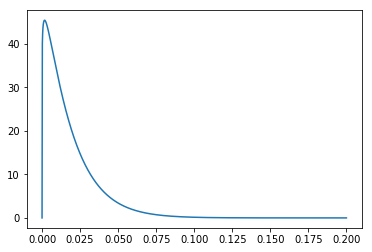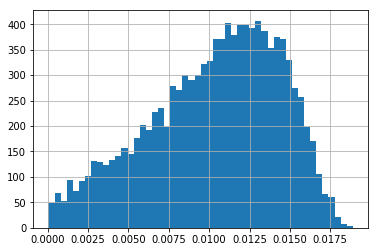# NaClhv

Theology, philosophy, science, math, and random other things

## Re-analyzing the Stanford COVID-19 antibody study

April 28, 2020

Re-analyzing the Stanford covid antibody study

Introduction and results

This is a re-analysis of Stanford's antibody study in Santa Clara County, which reported a population prevalence of 2.5% to 4.2% for COVID-19 antibodies, and a corresponding infection fatality rate of 0.12% to 0.2%. This result, if true, would have huge implications, as the lower fatality rate would dramatically change the calculus on important policy decisions, like when and how we should reopen the economy. However, this study has also received numerous criticisms, most notably for the results being inconsistent with the false positive rate of the antibody test.

Here, I attempt to derive what the results ought to have been, under a better methodology. I will be using a Bayesian approach, employing beta distributions to model probabilities.

The results I get at the end are as follows:

``Antibody prevalence in study participants: 1.0% (95% CI: 0.16% to 1.7%)Antibody prevalence in Santa Clara (speculative, due to missing data):1.9% (95% CI: 0.3% to 3.2%)Infection fatality rate implied from above:0.27% (95% CI: 0.17% to 1.6%)``

This fatality rate is quite uncertain on its own, but it is in broad agreement with other similar kinds of studies.

Methodology and code

Alright, let's begin. First, let's import some packages:

In :
`import numpy as npimport pandas as pdfrom scipy.stats import beta%matplotlib inline`
Next, let's decide on a prior for the prevalence of antibodies in the study:
In :
`p_prior = beta(1.1, 60)x = np.linspace(0, 0.2, 1000)pd.Series(p_prior.pdf(x), index=x).plot()`
Out:
`<matplotlib.axes._subplots.AxesSubplot at 0x1f908dd0c88>`In :
`p_prior.mean(), p_prior.median(), p_prior.interval(0.95)`
Out:
`(0.01800327332242226, 0.013074113173063773, (0.0006173369513848543, 0.06281995654253102))`

Note that this prior is quite favorable to the results of the study. It piles on the prior probability right on top of where the study's results turned out to be (1.5%), with the mean and the median of the distribution falling right into the interval cited by the study (1.1-2%). So we are making an assumption a priori that the results of the study are correct. Of course, in a good Bayesian analysis this doesn't matter much in the end, as the prior should get overwhelmed by the evidence from the data.

Next, let's model the specificity and sensitivity of the antibody test they used. Pooling together the numbers they provided in the paper, we get:

In :
`sensitivity = beta(78 + 25 + 0.5, 7 + 12 + 0.5)specificity = beta(30 + 369 + 0.5, 0 + 2 + 0.5)`
We next run a simulation using random samples from these beta distributions, then only look at the results which actually return the empirical results of the study. This satisfies Bayes rule, which says that the probability values which best predict the outcome should be favored. It's consistent with the adage that “when you have eliminated the impossible, whatever remains, however improbable, must be the truth”. It undergirds Bayesian hierarchical modeling, and it's the same methodology I used in my argument for the resurrection of Jesus Christ.

The data from the study says that there were 50 positive cases out of 3330.

In :
`n_sim = 1000000total_cases = 3330positive_cases = 50df = pd.DataFrame()df["p_population"] = p_prior.rvs(n_sim)df["sensitivity"] = sensitivity.rvs(n_sim)df["specificity"] = specificity.rvs(n_sim)df["detected_positives"] = (    # true positives    df["p_population"] * df["sensitivity"]     # plus false positives    + (1 - df["p_population"]) * (1 - df["specificity"])).apply(lambda x: round(x * total_cases))#"eliminate the impossible":data_df = df[df["detected_positives"] == positive_cases]data_df.head(3)`
Out:
p_populationsensitivityspecificitydetected_positives
810.0053930.9094200.98978950
2260.0034340.7811800.98762950
4550.0111680.8915030.99487650

The distribution of true prevalence of the antibodies can then simply be read off from the "p_population" column:
In :
`data_df["p_population"].hist(bins=50)`
Out:
`<matplotlib.axes._subplots.AxesSubplot at 0x1f9090fce80>`The relatively smooth distribution shows that the number of simulations was sufficient. Note that the distribution runs right up to 0, as you'd expect with a specificity with a lower bound of about 98%. The actual prevalence of antibodies in the study can then be characterized as follows:
In :
`data_df["p_population"].mean()`
Out:
`0.010314341450043712`

In :
`data_df["p_population"].quantile([0.025, 0.975])`
Out:
`0.025    0.0016080.975    0.016619Name: p_population, dtype: float64`

So we have an antibody prevalence of 1.0% (95% CI: 0.16% to 1.7%) for the study participants.
Discussion on demographic reweighing

This now needs to be re-weighed to match the demographics of Santa Clara county. The paper mentions that it re-weighed the samples by zip code, race, and sex.

In order to do this calculation, we would need every zip-race-sex combination in the study, along with the detected number of positives and negatives in that combination. Unfortunately, the paper doesn't provide that data - presumably for privacy reasons. Fair enough.

However, I will note that this re-weighing is highly unlikely to reduce the uncertainty in our metric. That is to say, if we had a perfectly random sample, then our study would perfectly reflect the population - and even so our uncertainty already spans a whole order of magnitude (0.16% to 1.7%). Could deviating from this ideal scenario make our final answer MORE certain? Any deviation, and the required adjustment, is far more likely to add uncertainty rather than certainty.

If I may engage in a bit of speculation here, I suspect this is where the study went wrong. As far as I can tell without the missing data, they calculated a point estimate for the prevalence in the study participants, then performed the reweighing to get the population-adjusted point estimate. This step nearly doubled the prevalence, from 1.5% to 2.8%. Meaning that, at this point, they were effectively thinking of the positives in their samples not as 50/3330, but 94/3330 - nearly doubling the number of positive samples artificially. Only after this adjustment did they correct for sensitivity and specificity - with the result that the inflated positive numbers were now able to outpace the false positive rate.

Now, it's not impossible for the correct procedure to end up doing something similar. After all, the individual demographic information is additional data, so adding in that data could add more certainty, and that certainty could work to increase the prevalence. But this would require a rare, specific set of circumstances, and very strong assumptions. So I'm not saying that the original paper was necessarily wrong - but I should like a release of the data itself, or at least a discussion of it, before I believed the results. Such rarities in the data would, in all likelihood, point to a flaw in the sampling rather than additional certainty.

And indeed there ARE flaws in the sampling, even apart from this issue. Two points stand out: first, the participants for the study were recruited via Facebook - which would naturally select for those who had higher reason to believe that they had been infected at some point. Second, the demographic combination mentioned above explicitly doesn't correct for age, which means that compared to the country, the study systematically underrepresents the elderly (65 or older). Of course, this is the demographic which has the most to lose from an infection, and so would be most cautious in trying not to catch it. So underrepresenting this group would cause the reported prevalence rate to be inflated.

Both of these effects would artificially increase the calculated prevalence rate - which means that we should be VERY cautious about the demographic reweighing further increasing our results. In particular, it is very difficult to justify the lower bound increasing from 0.16% in the study participants, to 2.5% in the population - which is the value that's given in the paper. Nor is that 2.5% lower bound from a proper 95% interval: it is rather just the smallest value among three constructed scenarios - where two of the scenarios have their own lower bounds BELOW this 2.5% value.

Results, comparison to other studies, and conclusion

Given all this, and the fact that the required data are simply not provided, perhaps the best we can do for the demographic reweighing is to simply increase our unweighed results proportionately, which would keep the relative uncertainty the same. In the study itself, the demographic reweighing increased the prevalence from 1.5% to 2.8% - a factor of 1.87. Doing the same to our results cited above gives:

Antibody prevalence of 1.9% (95% CI: 0.3% to 3.2%) for the Santa Clara County.

Using the same number of deaths as in the study (100), this translates to:

infection fatality rate of 0.27% (95% CI: 0.17% to 1.6%).

This is a tentative result obtained by fudging around the missing data, and the uncertainty range is broad and not particularly helpful for policy setting - and yet it provides some measure of insight in conjunction with other similar studies.

For example, There's a German antibody study that reports an infection fatality rate of 0.37% (with a 14% prevalence, which makes it robust against false positives).

An antibody test in New York gives a rate of 0.5% (with a 10-20% prevalence, again making it robust against false positives).

Preliminary results from two other antibody tests have also been released: USC conducted a test in LA county, with an infection mortality rate of 0.13% to 0.27%, and a prevalence rate of 4.1%. University of Miami conducted a study of the Miami-Dade county, which gave a prevalence rate of 4.4% to 7.9%. With some 300 deaths in the county, that translates to an infection mortality rate of 0.14% to 0.24%. These seem to share much of the same characteristics as the Santa Clara study: blood tests reporting low prevalence. In addition, they have smaller sample sizes. It's not yet known whether they share the same flaws.

A study for the Diamond Princes cruise ship gives an adjusted infection fatality rate of 0.6%, with a 95% CI of 0.2% to 1.3%.

A group of pregnant women were also tested in New York City. About 14% of them tested positive for the virus. This is an atypical demographic group who were tested with a different method, so their results cannot be expanded to the whole population. But the results here are roughly consistent with the previously cited New York study, reinforcing the 5% number.

Lastly, Iceland performed a sampling from their whole population, testing for the active virus itself. They reported that about 0.7% of their population actively had the virus in the 20 days leading up to April 4th. Given that their total cases number about 3 times the average active number they had during those dates, this roughly translates to a 2.1% prevalence rate and a 0.13%. But there are huge uncertainties associated with this number - it's not known how many tests were performed at which points in the infection's progress, and Iceland is a small country - they only have 10 deaths and a total population of 360 thousand people.

So looking at these previous results, we can say that our Santa Clara study, when re-analyzed as above, is in line with the rest. Though there are still large uncertainties, these studies seem to be converging roughly in the ballpark of 0.2% to 0.6% for the infection fatality rate.

You may next want to read:
Quick takes on the plan to re-open the country
Keeping score: my coronavirus predictions